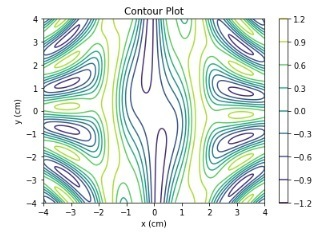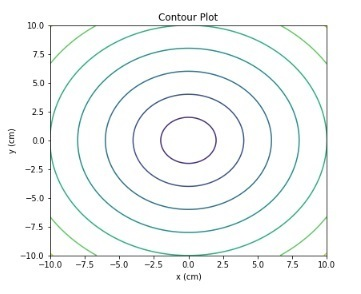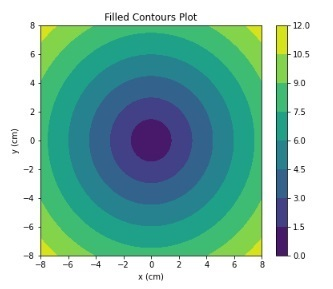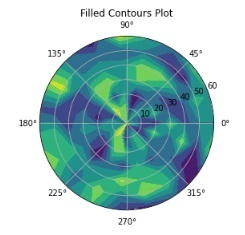# Contour Plot using Python Matplotlib

Matplotlib is free and open-source plotting library in python. It is used to create 2-Dimensional graphs and plots by using python scripts. To utilize the matplotlib functionalities we need to install the library first.

## Install using pip

By execute the below command in the command prompt we can easily install the latest stable package for Matplotlib from PyPi.

pip install Matplotlib


You can install Matplotlib through conda, using the following command –

conda install -c conda-forge matplotlib


A Contour plot is used for visualizing three-dimensional data in a two-dimensional surface by plotting constant z slices, called contours.

It is drawn with the help of a contour function (Z), which is a function of two inputs X, and Y (the X-axis and Y-axis coordinates).

Z = fun(x,y)


The Matplotlib provides two functions plt.contour, and plt.contourf to plot the contour plots.

## The contour() Method

The matplotlib.pyplot. contour() method is used to draw contour lines. It returns the QuadContourSet. Following is the syntax of this function –

contour([X, Y,] Z, [levels], **kwargs)


### Parameters

• [X, Y]: Optional parameter, it represents the coordinates of the values in Z.

• Z: The height values over which the contour is drawn.

• levels: It is used to determine the numbers and positions of the contour lines / regions.

### Example

Let’s take an example and plot the contour lines using the numpy trigonometric functions.

import numpy as np
import matplotlib.pyplot as plt

def f(x, y):
return np.sin(x) ** 10 + np.cos(10 + y * x) * np.cos(x)

xlist = np.linspace(-4.0, 4.0, 800)
ylist = np.linspace(-4.0, 4.0, 800)

# A mesh is created with the given co-ordinates by this numpy function
X, Y = np.meshgrid(xlist, ylist)
Z = f(X,Y)

fig = plt.figure()
ax = fig.add_axes([0.1, 0.1, 0.8, 0.8])

cp = ax.contour(X, Y, Z)
fig.colorbar(cp) # Add a colorbar to a plot
ax.set_title('Contour Plot')

ax.set_xlabel('x (cm)')
ax.set_ylabel('y (cm)')
plt.show()


### OutputThe f(x,y) function is defined using the numpy trigonometric functions.

### Example

Let’s take another example and draw contour lines.

import numpy as np
import matplotlib.pyplot as plt

def f(x, y):
return np.sqrt(X**2 + Y**2)

xlist = np.linspace(-10, 10, 400)
ylist = np.linspace(-10, 10, 400)

# create a mesh
X, Y = np.meshgrid(xlist, ylist)

Z = f(X, Y)

fig = plt.figure(figsize=(6,5))
ax = fig.add_axes([0.1, 0.1, 0.8, 0.8])

cp = ax.contour(X, Y, Z)
ax.set_title('Contour Plot')
ax.set_xlabel('x (cm)')
ax.set_ylabel('y (cm)')
plt.show()


### OutputThe z-function is the sum of the square root of x and y coordinate values. Implemented by using the numpy.sqrt() function.

## The contourf() function

The matplotlib.pyplot provides a method contourf() to draw filled contour. Following is the syntax of this function –

contourf([X, Y,] Z, [levels], **kwargs)


Where,

• [X, Y]: Optional parameter, it represents the coordinates of the values in Z.

• Z: The height values over which the contour is drawn.

• levels: It is used to determine the numbers and positions of the contour lines / regions.

### Example

Let’s take another example and draw the contour plot using the contourf() method.

import numpy as np
import matplotlib.pyplot as plt

xlist = np.linspace(-8, 8, 800)
ylist = np.linspace(-8, 8, 800)

X, Y = np.meshgrid(xlist, ylist)
Z = np.sqrt(X**2 + Y**2)

fig = plt.figure(figsize=(6,5))
ax = fig.add_axes([0.1, 0.1, 0.8, 0.8])

cp = ax.contourf(X, Y, Z)
fig.colorbar(cp) # Add a colorbar to a plot
ax.set_title('Filled Contours Plot')
#ax.set_xlabel('x (cm)')
ax.set_ylabel('y (cm)')
plt.show()


### OutputUsing fig.colorbar() method we have added the colours to the plot. The z function is the sum of the square root of x and y coordinates values.

### Example

In this example, we will plot a Polar contour plot using the matplotlib.plt.contourf() method.

import numpy as np
import matplotlib.pyplot as plt

b = np.arange(0, 70, 10)

Y, X = np.meshgrid(b, a)
values = np.random.random((a.size, b.size))

fig, ax = plt.subplots(subplot_kw=dict(projection='polar'))
ax.set_title('Filled Contours Plot')
ax.contourf(X, Y, values)

plt.show()


### OutputIn all the above examples we have used the numpy.meshgrid() function to produce arrays for X and Y coordinates.

Updated on: 30-May-2023

390 Views